Multivariable Calculus : Vector Equations

Example Questions

Example Question #5 : Multivariable Calculus

Write down the equation of the line in vector form that passes through the points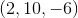, and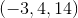.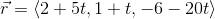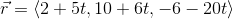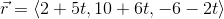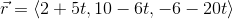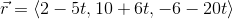Explanation:

Remember the general equation of a line in vector form: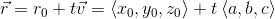, where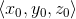is the starting point, and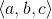is the difference between the start and ending points.

Lets apply this to our problem.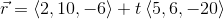Distribute the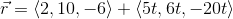Now we simply do vector addition to getExample Question #6 : Multivariable Calculus

Write down the equation of the line in vector form that passes through the points, and.Explanation:

Remember the general equation of a line in vector form:, whereis the starting point, andis the difference between the start and ending points.

Lets apply this to our problem.Distribute theNow we simply do vector addition to get